# Image deblurring using regularization

I'd like to welcome back guest blogger Stan Reeves, professor of Electrical and Computer Engineering at Auburn University, for another in his series of posts on image deblurring. -Steve

I've previously blogged about image restoration methods based on inverse filtering and Wiener filtering. Wiener filtering gives really impressive results compared to inverse filtering. However, Wiener filtering assumes that the image is modeled as a random process for which 2nd-order statistics are known, along with the noise variance. This viewpoint may not be attractive to some users, and the required information may not always be available.

Regularized restoration provides similar results to Wiener filtering but is justified by a very different viewpoint. Also, less prior information is required to apply regularized restoration. (Note: The Image Processing Toolbox function that implements regularized restoration is called deconvreg.)

As before, I will assume a shift-invariant blur model with independent additive noise, which is given by

y(m,n) = h(m,n)*x(m,n) + u(m,n)

where * is 2-D convolution, h(m,n) is the point-spread function (PSF), x(m,n) is the original image, and u(m,n) is noise.

Regularization trades off two desirable goals -- 1) the closeness of the model fit and 2) the closeness of the model behavior to something that would be expected in the absence of specific knowledge of the model parameters or data. Remember that inverse filtering minimizes: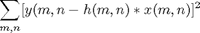which fits the model to the data as closely as possible.

As a reminder, let's look at the horrible results that this seemingly logical procedure can yield:

% form PSF as a disk of radius 3 pixels
h = fspecial('disk',4);
% read image and convert to double for FFT
hf = psf2otf(h,size(cam));
cam_blur = real(ifft2(hf.*fft2(cam)));
imshow(cam_blur)
title('blurred image')
xlabel('(original image courtesy Massachusetts Institute of Technology)')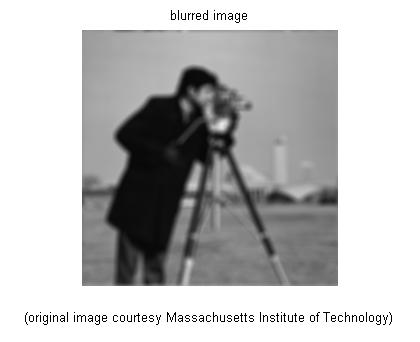sigma_u = 10^(-40/20)*abs(1-0);
cam_blur_noise = cam_blur + sigma_u*randn(size(cam_blur));
imshow(cam_blur_noise)
title('noisy image')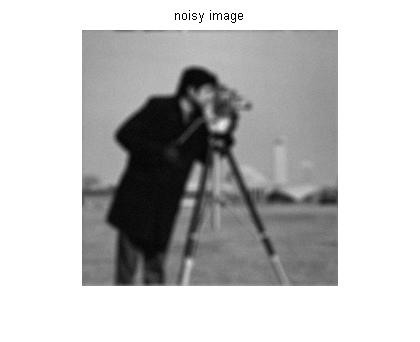cam_inv = real(ifft2(fft2(cam_blur_noise)./hf));
imshow(cam_inv)
title('inverse filter restoration')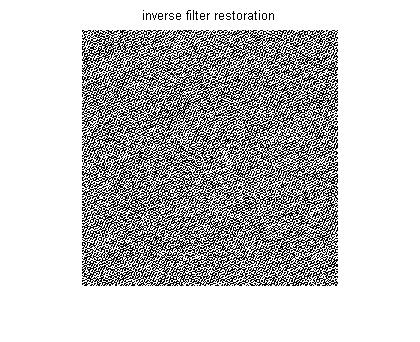The problem is that the solution fits the noise as well as the underlying data. The inverse filter divides by some very small values where noise in the numerator is relatively large compared to the attenuated signal. As a result, the solution becomes far too rough. Regularization adds another term to the minimization criterion to force the image to be somewhat smooth: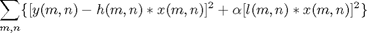The second term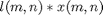is a filtered version of the estimated image that we expect to be small. In image processing, we usually expect a high level of local correlation or smoothness in the image. So the filter is chosen as a highpass filter so that we minimize the high-frequency content or "roughness" in the solution. The parametercontrols the degree of the roughness penalty. The larger it is, the smoother the restored image will be.

We can rewrite the minimization criterion in the DFT domain as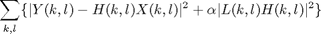Taking a derivative with respect to the image DFT coefficients and setting the result to zero yields the regularized restoration in the DFT domain: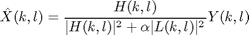The key to the performance of this filter is the extra term in the denominator. If this extra term is zero, the filter reverts to an inverse filter. However, for a non-zero term, the denominator is prevented from growing too small, especially at higher frequencies where the attenuated signal is likely to be quite small. Thus, noise amplification resulting from division by very small numbers is significantly reduced.

The regularization filter is often chosen to be a discrete Laplacian: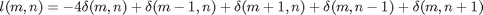The regularization parameter alpha can be chosen either by trial-and-error or by one of several different statistical methods. Some of these methods require no additional prior information.

lf = fft2([0 1 0; 1 -4 1; 0 1 0],256,256);
alpha = 0.01;
cam_inv = real(ifft2(fft2(cam_blur_noise)./hf));
cam_reg = deconvreg(cam_blur_noise,h,0,alpha);

imshow(cam_reg)
title('regularized filter restoration')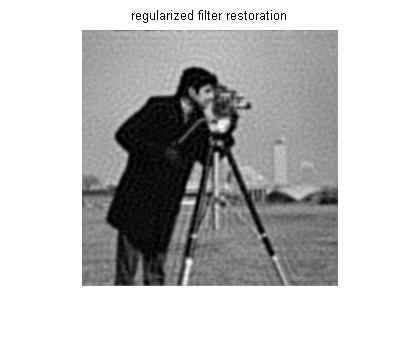This filter can be understood as an approximation of a Wiener filter. If we choose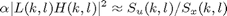then the result approximates the Wiener filter expression.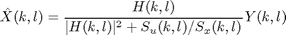Let's explore the role of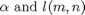alpha = 0.0005;
cam_reg = deconvreg(cam_blur_noise,h,0,alpha);
imshow(cam_reg)
title('\alpha = 0.0005')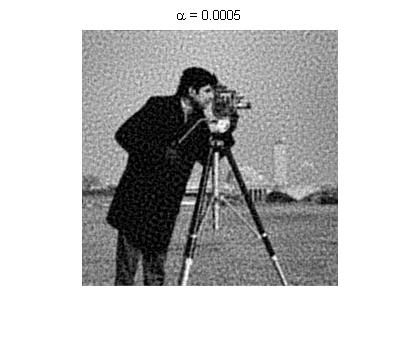alpha = 0.01;
cam_reg = deconvreg(cam_blur_noise,h,0,alpha);
imshow(cam_reg)
title('\alpha = 0.01')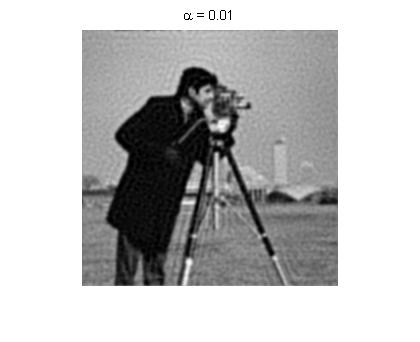alpha = 0.2;
cam_reg = deconvreg(cam_blur_noise,h,0,alpha);
imshow(cam_reg)
title('\alpha = 0.2')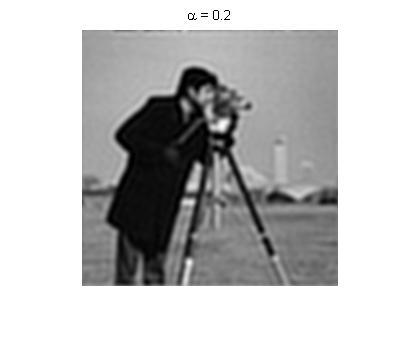It is evident from the images that a smaller alpha results in a noisier but sharper image while larger alpha results in a cleaner but blurrier image.

While the choice of the regularization filter does have an influence on the results, even a non-optimal choice can yield good results. If we use an impulse (no filtering) instead of the high-pass discrete Laplacian, we get the following results:

alpha = 0.01;
regop = 1;
cam_reg = deconvreg(cam_blur_noise,h,0,alpha,regop);
imshow(cam_reg)
title('l(m,n) = no filtering')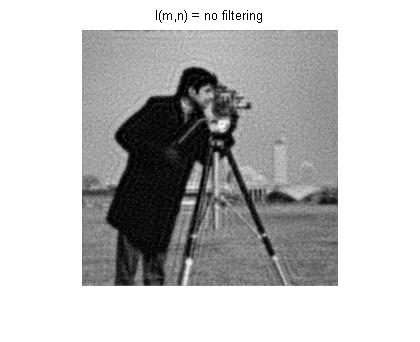alpha = 0.01;
lf = fft2([0 1 0; 1 -4 1; 0 1 0],256,256);
cam_reg = deconvreg(cam_blur_noise,h,0,alpha);
imshow(cam_reg)
title('l(m,n) = discrete Laplacian')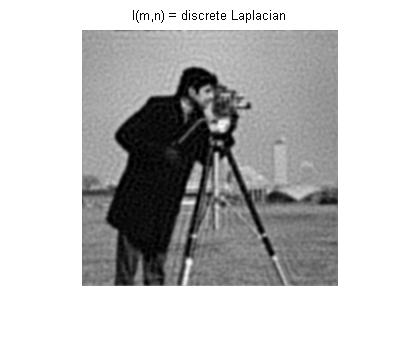The results are pretty insensitive to the type of filter used to penalize roughness. Even no filtering (penalizing only the sum of squared pixel values) yields results that are nearly as good as the discrete Laplacian.

Regularization can be a useful tool when statistical information is unavailable. Furthermore, this framework can be extended to adapt to image edges, spatially varying noise, and other challenges. But those are topics for other blogs...

- by Stan Reeves, Department of Electrical and Computer Engineering, Auburn University

Published with MATLAB® 7.6

|